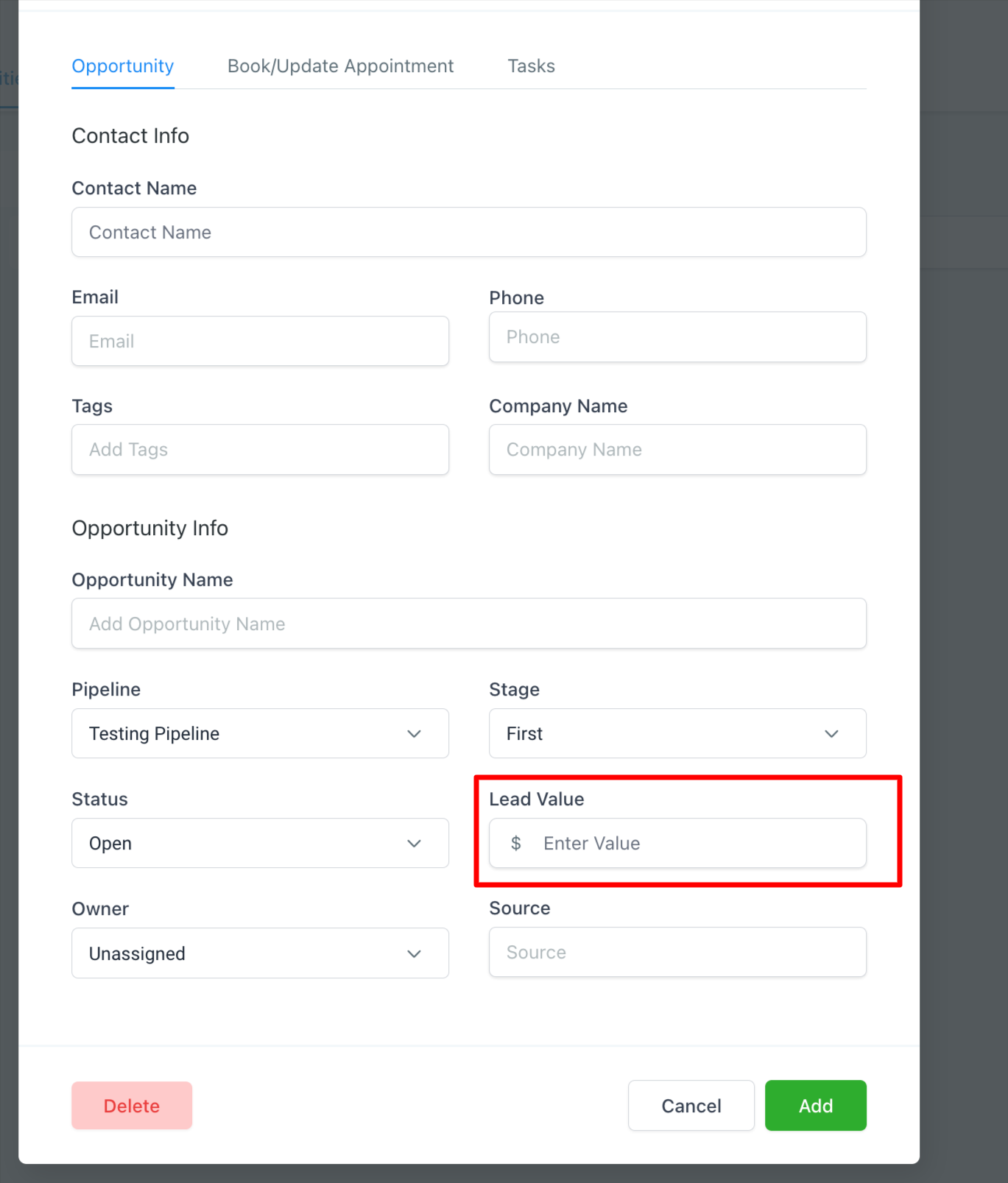## 1. Impressions

An impression is the number of times your ad is displayed on a search results page or another website within the Google network.

## 4. Client Spend

Is the cost of running the ad plus the management fees (this is only visible to Agency Admin and Agency User)

## 5. Average CPC (Cost per Click)

CPC is the average amount that a publisher pays for each click on their ad. The higher the CPC, the more expensive it will be to place an advertisement.

The cost of placing an advertisement can vary depending on many factors such as:

• Number of impressions
• Location and time of day
• Type of content in which

## 6. Cost Per Conversion (CPA)

is a metric that measures the average cost of acquiring one new customer. It’s also known as Cost per Acquisition, or CPA. It is calculated by dividing the total cost of ads by the total number of conversions.

## 7. Conversion Rate

Conversion rates are calculated by simply taking the number of conversions and dividing that by the number of total ad interactions that can be tracked to a conversion during the same time period. For example, if you had 50 conversions from 1,000 interactions, your conversion rate would be 5%, since 50 ÷ 1,000 = 5%

## 8. Revenue

When an opportunity is marked as won, the opportunity will have a Lead value (monetary) added. It is sum total of all the opportunity which is marked as won for a specific google ad campaign.

`When adding a lead value only use numerical integers. There is no need to add a \$ sign as its already accounted for in the field (See example below)`## 9. ROI

Return On Investment (ROI) is measured by calculating the ratio between the costs incurred during an advertising campaign and the revenues generated from that campaign. ROI can be applied to each individual ad, or to the entire page.

## 10. Sales

It refers to the leads/opportunities that have been marked as won.## 11. CPS (Cost per Sales)

CPS is measured by dividing the cost of running a specific campaign by the number of opportunities marked as won.